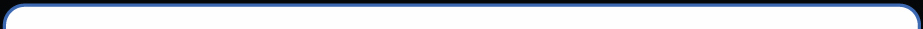Speaker Crossover Calculators

Ohms

Ohms

Hz
C1= µF
L1= mH

Ohms

Ohms

Hz
C1= µF
L1= mH
C2= µF
L2= mH

Ohms

Ohms

Hz
C1= µF
C2= µF
L1= mH
L2= mH
L3= mH
C3= µF

Ohms

Ohms

Hz
C1= µF
C2= µF
L1= mH
L2= mH
C3= µF
C4= µF
L3= mH
L4= mH

Zobel Circuit (Impedance Stabilization)

Ohms

Henries
C1= µF
R1= Ohms